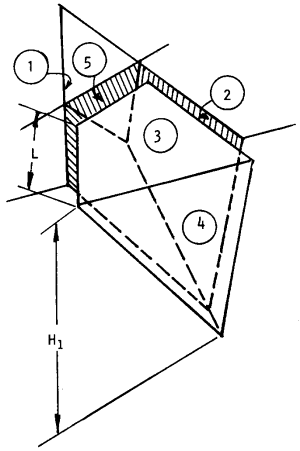The Rocscience International Conference 2021 Proceedings are now available. Read Now

# Slope Height

The Slope Height in SWedge is defined as the VERTICAL distance from the base of the slope to the crest of the slope (i.e. the line of intersection of the two slope planes).

If the crest of the slope is not horizontal, the Slope Height is measured from the point of intersection of Joint plane 1 with the crest. This is shown as distance H1 in the following figure.

The Slope Height is always required input data for an SWedge model, and determines the maximum wedge size which can be formed for a given set of joint orientations. The Slope Height is entered in the Input Data dialog.

Arbitrary wedge illustrating the definition of Slope Height (H1).